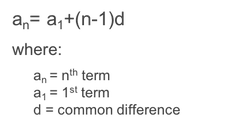sequences
arithmetic sequenceCommon Difference Next Term N-th Term Value given Index Index given Value SumSequence
Sequence
Sequence
Sequence
Index
Sequence
Value
Sequence
Start index
End indexarithmetic-sequence-calculator

en